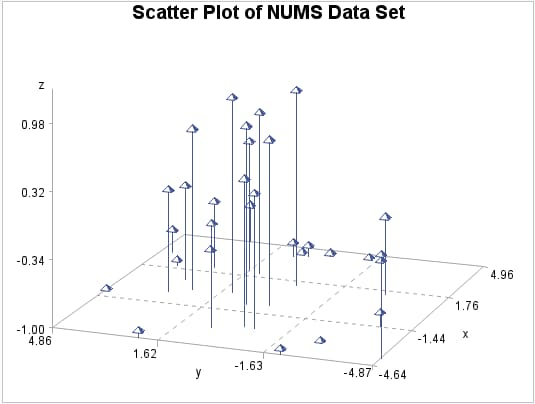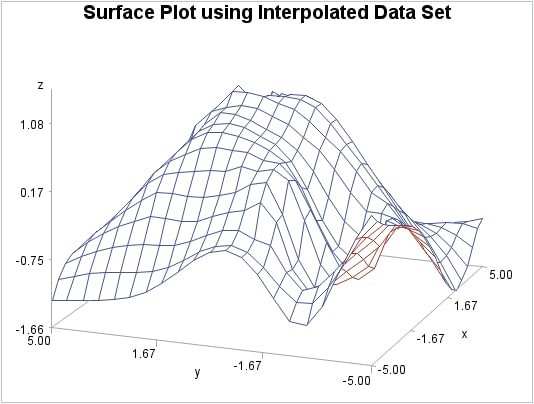Example 1: Using the Default Interpolation Method

 Features: G3GRID statement option OUT= GRID statement options AXIS1= and AXIS2= Other features: DATA step and the G3D procedure Sample library member: GTGDEFIN
This example demonstrates the default interpolation method that is used by the GRID statement. The example first generates a scatter plot of random data to show the concentration of data values before processing the data set with the G3GRID procedure. The original data does not contain enough combinations of x, y, and z values to generate a surface plot with the G3D procedure or generate a contour plot with the GCONTOUR procedure.
Scatter Plot of NUMS Data Set (gtgdefin)The example then runs the G3GRID procedure to interpolate additional x, y, and z values. Because no interpolation method is specified, the default interpolation method is used. The resulting output data set is used as input to the G3D procedure, which generates the surface plot shown in the following output.
Surface Plot Using Interpolated Data Set (gtgdefin)Program

`goptions reset=all border;`
```data nums;
keep x y z;
do i=1 to 30;
x=10*ranuni(33)-5;
y=10*ranuni(35)-5;
z=sin(sqrt(x*x+y*y));
output;
end;
run;```
`title "Scatter Plot of NUMS Data Set";`
```proc g3d data=nums;
scatter y*x=z;
run;
quit;```
```proc g3grid data=nums out=default;
grid y*x=z /
axis1=-5 to 5 by .5
axis2=-5 to 5 by .5;
run;
quit;```
```title "Surface Plot using Interpolated Data
Set";```
```proc g3d data=default;
plot y*x=z;
run;
quit;```

Program Description

Set the graphics environment.
`goptions reset=all border;`
Create data set. NUMS uses a set of randomly sampled points to create the data used in this, and all remaining examples in this chapter.
```data nums;
keep x y z;
do i=1 to 30;
x=10*ranuni(33)-5;
y=10*ranuni(35)-5;
z=sin(sqrt(x*x+y*y));
output;
end;
run;```
Define the title for the plot.
`title "Scatter Plot of NUMS Data Set";`
Generate the scatter plot with PROC G3D.
```proc g3d data=nums;
scatter y*x=z;
run;
quit;```
Grid the data with PROC G3GRID. The OUT= option on PROC G3GRID specifies a name for the temporary output data set. The GRID option specifies the variables Y*X=Z for the output data set. The AXIS statements define axes ranges.
```proc g3grid data=nums out=default;
grid y*x=z /
axis1=-5 to 5 by .5
axis2=-5 to 5 by .5;
run;
quit;```
Define the title for the plot.
```title "Surface Plot using Interpolated Data
Set";```
Generate the surface plot. The G3D procedure using the G3GRID procedure's output data set as the input data set.
```proc g3d data=default;
plot y*x=z;
run;
quit;```